## Section7.10Momentum Bootcamp

### Subsection7.10.9Miscellaneous

Two blocks of masses $m_1$ and $m_2$ separated by a horizontal distance $D$ are dropped from the same height $h$ at the same time. Find the vertical position of the center of mass at a time $t$ before the two blocks strike the ground. Assume no air resistance.

Hint

Free fall of Center of Mass.

$Y_\text{cm} = h -\dfrac{1}{2}\, g\, t^2\text{.}$

Solution

We use positive $y$ coordinate pointed up with origin at the ground. Since only external force on the two blocks is the gravity, we have a free-fall motion of the center of mass. This will give the following equation for the $Y_\text{cm}$ at instant $t \text{.}$

\begin{equation*} Y_\text{cm} = Y_\text{cm}(0) + V_\text{cm}(0)\, t + \dfrac{1}{2}\, a_y\, t^2. \end{equation*}

Here $a_y = - g \text{,}$ $V_\text{cm}(0) = 0 \text{,}$ and

\begin{equation*} Y_\text{cm}(0) = \dfrac{m_1 h + m_2 h}{m_1 + m_2} = h. \end{equation*}

Therefore,

\begin{equation*} Y_\text{cm} = h -\dfrac{1}{2}\, g\, t^2. \end{equation*}

Two particles of masses $m_1$ and $m_2=\dfrac{m_1}{2}$ move uniformly in different circles of radii $R_1$ and $R_2$ about origin in the $xy$ plane. The $x$ and $y$ coordinates of the center of mass and that of particle 1 are given as follows where length is in meters and $t$ in seconds.

\begin{align*} \amp X_{\text{cm}} (t) = 3\cos(2\,t),\ \ Y_{\text{cm}} (t) = 3\sin(2\,t),\\ \amp x_1 (t) = 4\cos(2\,t),\ \ y_1 (t) = 4\sin(2\,t). \end{align*}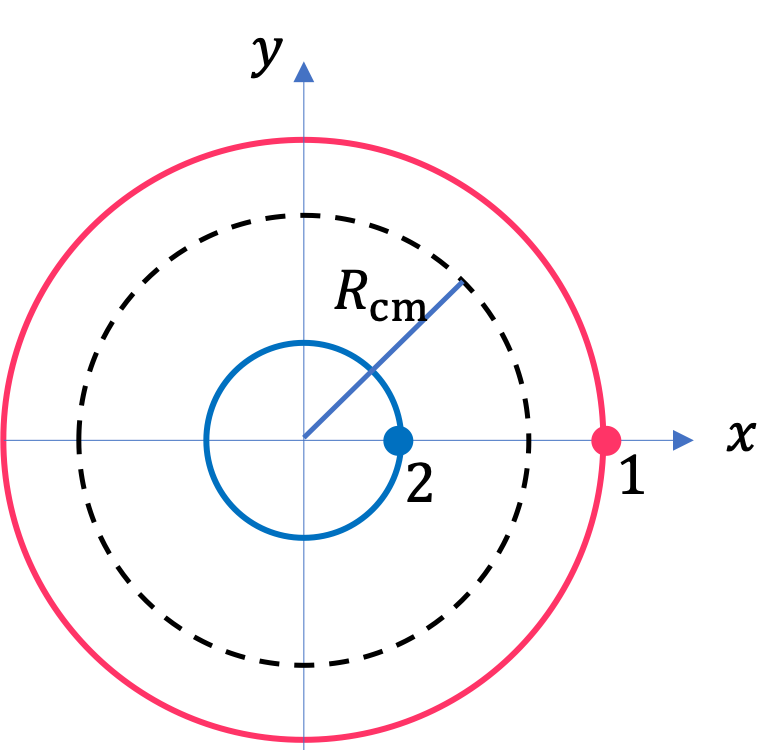(a) Find the radius of the circle in which the particle 1 moves.

(b) Find the $x$ and $y$-coordinates of particle 2 and the radius of the circle this particle moves.

Hint

(a) Use equation of a circle, (b) Use definition of $X_\text{cm}$ and $Y_\text{cm}\text{.}$

(a) $4\text{ m}\text{,}$ (b) $x_2 = 1.0\,\cos(2t) \text{,}$ $y_2 = 1.0\,\sin(2t)$ , $1.0\,\text{ m}\text{.}$

Solution 1 (a)

(a) From the equation of a circle, we get the radius $r_1$ from $x_1$ and $y_1\text{.}$ Let's use $\theta = 2\, t\text{.}$

\begin{equation*} r_1 = \sqrt{ x_1^2 + y_1^2 } = 4\sqrt{ \cos^2 \theta + \sin^2 \theta } = 4. \end{equation*}
Solution 2 (b)

(b) We use the definition of $X_\text{cm}$ to solve for $x_2\text{.}$ Let's write $M$ for $m_1 + m_2 \text{.}$

\begin{equation*} M\,X_\text{cm} = m_1 x_1 + m_2 x_2 \end{equation*}

gives

\begin{equation*} x_2 = \dfrac{1}{m_2} \left( M\,X_\text{cm} - m_1 x_1 \right). \end{equation*}

Now, we use the given information to get

\begin{equation*} x_2 = \dfrac{2}{m_1} \left( 1.5\, m_1\, 3\cos(2\,t) - m_1\, 4\cos(2\,t) \right) = 1.0\, \cos(2\,t). \end{equation*}

Similarly, we will get

\begin{equation*} y_2 = 1.0\, \sin(2\,t). \end{equation*}

From $x_2$ and $y_2\text{,}$ we get the radius of the circle in which 2 moves.

\begin{equation*} r_2 = 1.0. \end{equation*}

Find the center of mass of a one-meter long rod, made of $50\text{-cm}$ long iron of density $8\text{ g/cc}$ and $50\text{ cm}$ long aluminum of density $2.7\text{ g/cc}$ rods.Hint

First replace each rod by a point mass at the center.

$12.4\text{ cm}$ from center towards the iron rod.

Solution

Let subscript 1 denote iron and 2 the aluminum. Let $A$ be the area of cross-sections of the rods, $L_1$ and $L_2$ their lengths, and $\rho_1$ and $\rho_2$ their densities. The masses of each rod are

\begin{align*} \amp m_1 = \rho_1 A L_1 = 400\, A, \\ \amp m_2 = \rho_2 A L_2 = 135\, A, \end{align*}

in units of $\text{grams}$ if $A$ is in $\text{cm}^2\text{.}$ We leave the units alone since they will cancel out in the end giving us the answer in the same unit as the unit of $L\text{.}$

Replacing each rod at the center by its mass by point masses we get a system of two point masses. Let us use $x$ axis that has origin at the center of the iron rod and the positive $x$ axis towards the aluminum rod.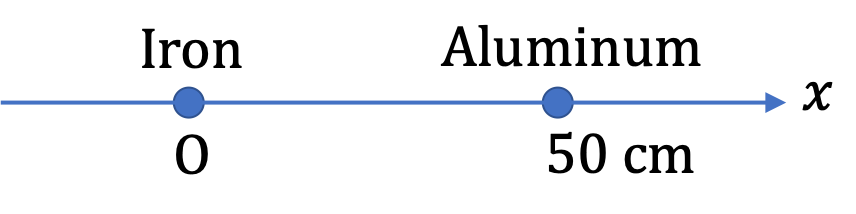This gives the point masses at $x_1=0$ and $x_2 = 50\text{ cm}\text{.}$ Hence $X_\text{cm}$ is

\begin{equation*} X_\text{cm} = \dfrac{400\, A \times 0 +135\, A\times 50 }{535\, A} = 12.6\text{ cm}. \end{equation*}

We can write this also as $25-12.6 = 12.4\text{ cm}$ from the center towards the iron rod.

A bullet of mass $200\text{ g}$ traveling horizontally towards East with speed $400\text{ m/s}$ strikes a block of mass $1.5\text{ kg}$ at rest on a frictionless table. After striking the block the bullet is imbedded in the block and the block and the bullet move together as one unit.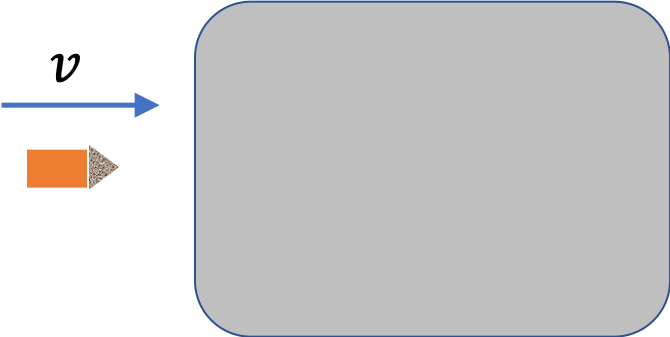(a) What is the magnitude and direction of the velocity of the block/bullet combination immediately after the impact?

(b) What is the magnitude and direction of the impulse by the block on the bullet.

(c) What is the magnitude and direction of the impulse from the bullet on the block?

(d) If it took $100\text{ msec}$ for the bullet to change the speed from $400\text{ m/s}$ to the final speed after impact, what is the average force between the block and the bullet during this time?

Hint

(a) Use conservation of momentum. (b) $J_\text{on bullet} = \Delta p_\text{bullet}\text{.}$ (c) $\vec J_\text{on block}^{by bullet} = -\vec J_\text{on bullet}^{by block} \text{.}$ (d) Use $F = J/\Delta t\text{.}$

(a) $47\text{ m/s}$ in the original direction of the bullet; (b) $70.6\text{ N.s}\text{,}$ towards bullet; (c) $70.6\text{ N.s}\text{,}$ towards block; (d) magnitude: $706\text{ N}\text{.}$

Solution 1 (a)

(a) Let $x$ be the direction of the motion of the bullet before collision as shown in Figure 7.10.51. Since block is at rest, the resulting motion will also be towards the positive $x$ axis. The conservation of $x$ component of the momentum gives us the following equaiton for the speed $V$ of the bullket/block combination after the collision.

\begin{equation*} 0.2\times 400 = \left( 0.2 + 1.5\right)\, V. \end{equation*}

This gives

\begin{equation*} V = 47.1\text{ m/s}. \end{equation*}
Solution 2 (b)

(b) We now look at the motion of the bullet by itself to determine the change in momentum of the bullet since the impulse on the bullet will equal the change in the momentum of the bullet. The simplified situation is shown in Figure 7.10.52.

Let us denote bullet by subscript 1 and the block by the subscript 2. Using the same coordinate system we get

\begin{equation*} J_x^\text{on 1} = p_{1x,f} - p_{1x,i} = 0.2\times 47.1 - 0.2\times 400 = -70.6 \text{ N.s}. \end{equation*}

Therefore, we find that the impulse on the bullet has magnitude $70.6 \text{ N.s}$ and has the direction opposite to its original velocity.

Solution 3 (c)

(c) We do not need to do any work for this part since by the third law of motion, the answer of this part will be negative of the answer for part (b). That is, the impulse on the block has magnitude $70.6 \text{ N.s}$ and has the direction same as the direction of the bullet's original velocity.

Solution 4 (d)

(d) From the impuse and duration, we get the magnitude of the average force between the block and the bullet to be

\begin{equation*} F_\text{av} = \dfrac{J}{\Delta t} = \dfrac{70.6 \text{ N.s}}{ 0.100 \text{ s}} = 706 \text{ N}. \end{equation*}

A billiard ball, labeled 1, moving horizontally strikes another ball, labeled 2, at rest at a glancing angle. Before the impact, ball 1 was moving at a speed of $3.0\text{ m/s}$ and after the impact it is moving at $1.0\text{ m/s}$ at $150^{\circ}$ from the original direction. If the two balls have equal masses, what is the velocity of ball 2 after the impact.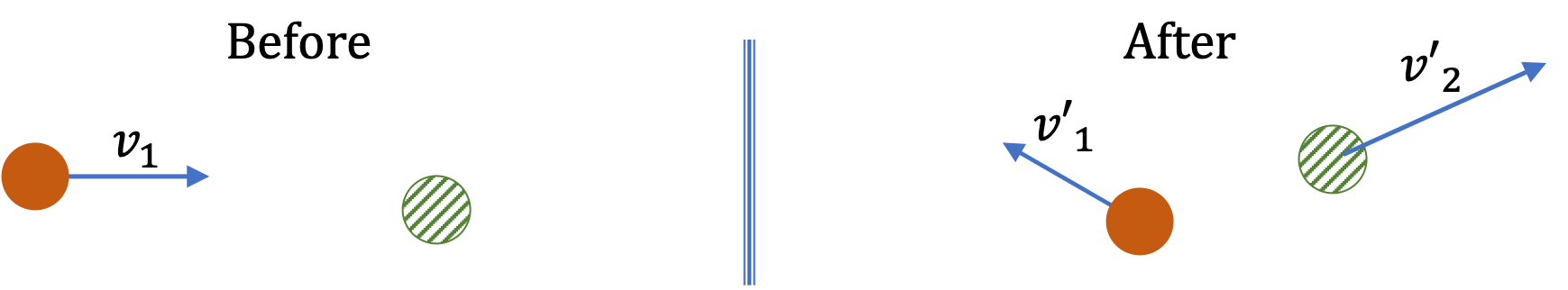Hint

Apply conservation of momentum along the two axes. Mass will cancel out.

$3.93\text{ m/s}\text{,}$ $7.4^{\circ}$ clockwise from the original direction.

Solution

To find the magnitude and direction of the velocity of the second ball after impact we will work out its $x$ and $y$ components. Refer to Figure 7.10.54 for the axes.

The conservation of momentum along the two axes gives us the following two equations, where we are omitting the mass since they will cancel out. We will write the initial values in each direction on the left side of the equation.

\begin{align*} 3.0 \amp = 1.0\,\cos(150^\circ) + v_{2x}^{\prime}\\ 0.0 \amp = 1.0\,\sin(150^\circ) + v_{2y}^{\prime} \end{align*}

Therefore, we have

\begin{align*} v_{2x}^{\prime} \amp = 3.9\text{ m/s}, \\ v_{2y}^{\prime} \amp = - 0.5\text{ m/s}. \end{align*}

This gives the magnitude and arc-tangent-angle as

\begin{gather*} v_{2}^{\prime} = \sqrt{3.9^2 + 0.5^2 } = 3.93\text{ m/s}, \\ \theta = \tan^{-1}\left(\dfrac{-0.5}{3.9} \right) = -7.3^{\circ}. \end{gather*}

Since $(3.9, -0.5)$ is in the fourth quadrant, the direction is $7.3^{\circ}$ clockwise from the positive $x$ axis.

Two projectiles of mass $m_1$ and $m_2$ with $m_2 \lt m_1$ are fired in the opposite directions from two launch sites as shown in Figure 7.10.56. The two launch sites are separated by a distance $D\text{.}$ The projectiles have the same initial speed and same angle with the horizon so that both reach the same spot in their highest point and strike there. As a result of the impact they stick together and move as a single body afterwards. Find the place they will land.

Hint

This problem has two parts: (i) the one-dimensional collision at the highest point, and (ii) a projectile motion afterwards.

$\left(\dfrac{m_1 D}{m_1 + m_2} \right)$ from the launch sire of $m_1\text{.}$

Solution

Strategy. This problem has two parts: (i) the one-dimensional collision at the highest point, and (ii) a projectile motion afterwards. We will use the axes shown in the figure below.

(i)The momentum conservation part. Let $v_0$ be the initial speed and $\theta$ the initial direction. Let the projetile 1 be initially located at the origin and the positive $x$ axis be towards projectile 2. his gives us the following for the constant $x$ velocities of the two projectiles.

\begin{align*} v_{1i,x} \amp = v_0\cos\,\theta,\\ v_{2i,x} \amp = -v_0\cos\,\theta = -v_{1i,x}. \end{align*}

After they collide, let $V_x$ be the $x$ component of the velocity of the two together. Conservation of momentum along the $x$ axis gives us

\begin{equation*} V_x = \dfrac{ m_1 v_{1i,x} + m_2 v_{2i,x}}{m_1 + m_2} = \dfrac{m_1 - m_2}{m_1 + m_2}\, v_{1i,x}. \end{equation*}

The projectile motion part. If we know the height at which this projectile starts, we can figure out the place it will land. To find the height we note that during the time 1 moved a distance $D/2\text{,}$ the max height $H$ was reached, where $v_{1y}=0\text{.}$ That is,

\begin{equation*} 0 = v_{1i,y}^2 -2gH, \text{ where } v_{1i,y} = v_0\sin\, \theta. \end{equation*}

This gives

\begin{equation*} H = \dfrac{v_0^2\sin^2\, \theta}{2g}. \end{equation*}

Now, we can use $V_x$ and $H$ to get the final point $(x, 0)$ from starting place, which is at $x_i=D/2$ and $y_i=H$ of the combined projectile. We get $t$ from the $y$ equation,

\begin{equation*} H = \dfrac{1}{2}gt^2, \end{equation*}

which gives

\begin{equation*} t = \sqrt{\dfrac{2H}{g}}. \end{equation*}

Using this in the $x$ equation gives the final $x$ coordinate.

\begin{equation*} x - \dfrac{D}{2} = V_x t = \dfrac{m_1 - m_2}{m_1 + m_2}\, v_{1i,x}\, \times \sqrt{\dfrac{2H}{g}}. \end{equation*}

Now we use $v_{1i,x}$ and $H$ and simplify the resulting expression. We get

\begin{equation*} x - \dfrac{D}{2} = \left(\dfrac{m_1 - m_2}{m_1 + m_2} \right)\dfrac{v_0^2\sin\,\theta\cos\,\theta}{g}. \end{equation*}

This expression has unknown $v_0$ and $\theta\text{.}$ Actually, their combination $v_0^2\sin\,\theta\cos\,\theta$ is related to the range $D$ of the original projectiles, since from the symmetry we can tell that if we had launched only projectile 1, it would land at a distance $D$ where the second site is located. Working out the range gives us (this is another projectile motion problem that you would need to work out.)

\begin{equation*} D = \dfrac{2v_0^2\sin\,\theta\cos\,\theta}{g}. \end{equation*}

Using this information, we get our final answer in the given quantities.

\begin{equation*} x = \dfrac{D}{2} + \left(\dfrac{m_1 - m_2}{m_1 + m_2} \right)\, \dfrac{D}{2} = \dfrac{m_1 D}{m_1 + m_2}. \end{equation*}

Grains from a grain hopper falls at a rate of $10\text{ kg/sec}$ vertically onto a freight car that is moving horizontally at a constant speed $2\text{ m/s}$ on a straight track. What force is needed to keep the freight car moving at a constant velocity.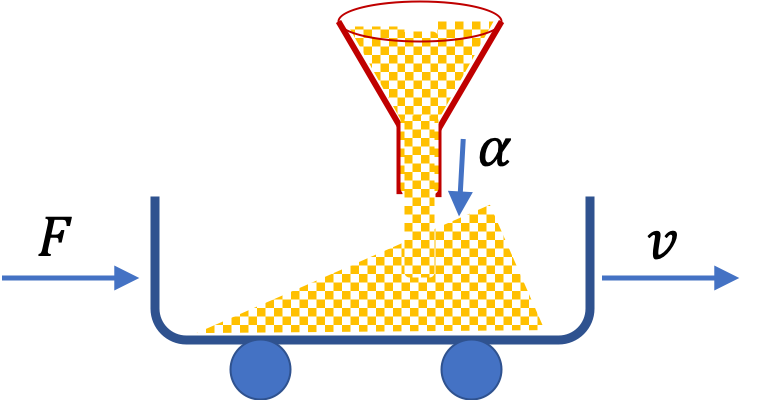Hint

Use Newton's law in change in momentum/impulse form along horizontal direction.

$20\text{ N}\text{,}$ horizontal in the direction of the velocity.

Solution

We will use Newton's law in change in momentum/impulse form along the $x$ axis. Let positive $x$ axis be pointed in the direction of the velocity of the freight car as shown in the figure.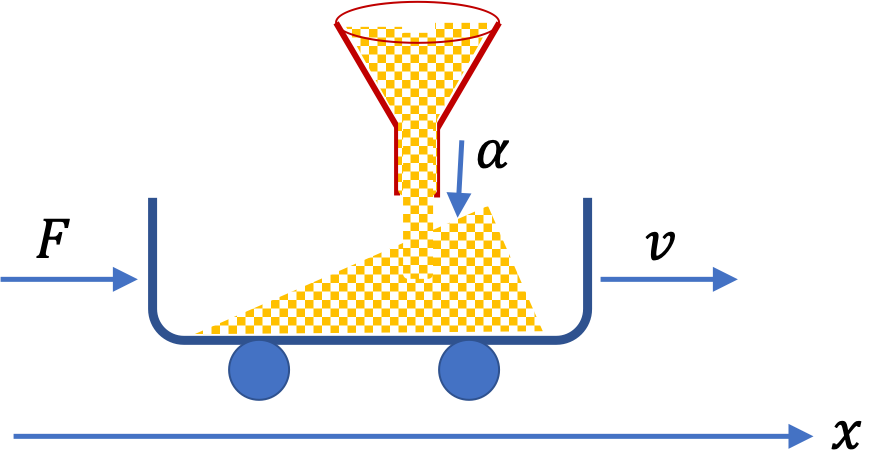Let us look at the change in $x$ component of momentum during an interval $t$ to $t+\Delta t\text{.}$ Let $\Delta m$ denote the amount of additional grain into the car during this time.

\begin{equation*} F\Delta t = (m + \Delta m) v - m v. \end{equation*}

Therefore, we get

\begin{equation*} F \Delta t = v\, \Delta m. \end{equation*}

Now, if grain is pouring in at the rate $\alpha \text{ kg/s}\text{,}$ then we will have

\begin{equation*} \Delta m = \alpha\Delta t. \end{equation*}

Hence, we get the following for the magnitude of the horizontal force needed to maintain the constant velocity of the car.

\begin{equation*} F = v\, \alpha. \end{equation*}

Using the numerical values given we get $F = 20\text{ N}\text{.}$ The direction of this force is, of course, in the direction of the velocity of the car.

A cube of side $a$ is cut out of another cube of side $b$ as shown in the figure below. Find the location of the CM of the structure. Hint: Think of the missing part as a negative mass overlapping a positive mass.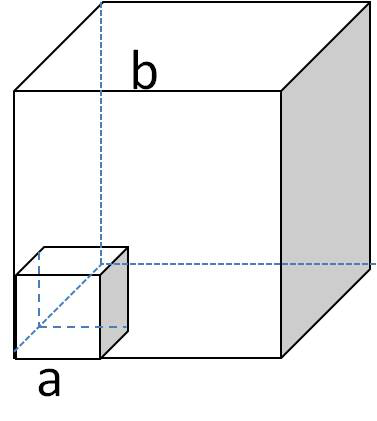Hint

Solution

Since center of mass comes from adding the mass-weighted coordinates, a positive mass and a negative mass at the same point in space will just add zero in the calculation. To figure out how much mass to place at the center of the empty space, let us construct the original cube with material from the corner missing by a sum of a full cube of density $\rho$ and a fake cube of density $-\rho$ placed in the corner space.

This gives two-point-mass problem, $m_1 = b^3\rho$ at $\left(\dfrac{b}{2}, \dfrac{b}{2}, \dfrac{b}{2} \right)\text{,}$ and another $m_2 = -a^3\rho$ at $\left(\dfrac{a}{2}, \dfrac{a}{2}, \dfrac{a}{2} \right)$ in a coordinate system with the origin at the corner and the axes along the edges. Therefore, the CM is located at

\begin{align*} X_\text{cm} \amp = \dfrac{1}{b^3-a^3} \left( \dfrac{b^4}{2} - \dfrac{a^4}{2} \right), \\ Y_\text{cm} \amp = X_\text{cm},\\ Z_\text{cm} \amp = X_\text{cm}. \end{align*}

A tennis ball machine has a number of tennis balls in it and rests on a fricionless table. The machine together with balls has mass $M\text{,}$ with each ball's mass $m\text{.}$ The machine shoots a ball in the forward direction at speed $u$ with respect to the machine at angle $\theta$ with horizon.

As a result, the machine recoils with a speed $V_1$ with respect to the table in the backward direction. While the machine is moving, it shoots the second ball which comes out at the same speed with respect to the machine, but now machine is moving at speed $V_2$ with respect to the table. (a) Find (a) $V_1$ and (b) $V_2\text{.}$ (c) Write a formula for the speed of the ball machine after $N$ balls have been shot.

Hint

Work with the $x$ axis of a coordinate system fixed to the ground and express all velocities with respect to this frame.

(a) $\dfrac{m}{M}\, u\,\cos\,\theta\text{,}$ (b) $\dfrac{m}{M-m}\, u\,\cos\,\theta\text{,}$ (c) $\dfrac{M u \cos\theta}{M-(N-1)m}\text{.}$

Solution 1 (a)

(a) We will use a coordinate system that is fixed to the ground and express all velocities with respect to this system. We will work with $x$ coordinate only with the positive $x$ axis in the direction in which the ball comes out. We will also denote quantities for machine with a subscript $1$ and that of a ball by subscript $2\text{.}$

The $x$-components of the velocities before and after the firing of the first ball with respect to the table are:

\begin{align*} \amp \text{Before: }\ \ v_{1x,i} = 0,\ \ v_{2x,i} = 0 \\ \amp \text{After: }\ \ v_{1x,f} = -V_1,\ \ v_{2x,f} = u\,\cos\,\theta - V_1 \end{align*}

Setting up the momentum conservation along $x$-axis we get

\begin{equation*} M\times 0 = (M-m) (-V_1) + m (u\,\cos\,\theta - V_1). \end{equation*}

Therefore,

\begin{equation*} V_1 = \dfrac{m}{M}\, u\,\cos\,\theta. \end{equation*}
Solution 2 (b)

Now, the velocity of the ball with respect to the table needs to be adjusted due to the machine moving at speed $V_2\text{.}$

\begin{align*} \amp \text{Before: }\ \ v_{1x,i} = -V_1,\ \ v_{2x,i} = u\,\cos\,\theta-V_1 \\ \amp \text{After: }\ \ v_{1x,f} = -V_2,\ \ v_{2x,f} = u\,\cos\,\theta-V_2 \end{align*}

Setting up the momentum conservation along the $x$ axis we get

\begin{equation*} (M-m)(-V_1) + m( u\,\cos\,\theta-V_1 ) = (M-2m)(-V_2) + m( u\,\cos\,\theta-V_2 ). \end{equation*}

Solving for $V_2$ and simplifying we get

\begin{equation*} V_2 = \frac{M}{M-m}V_1 = \dfrac{m}{M-m}\, u\,\cos\,\theta. \end{equation*}
Solution 3 (c)

The results of (b) shows us how the process will generalize to

\begin{equation*} V_N = \frac{M u \cos\theta}{M-(N-1)m}. \end{equation*}

A $65$-kg person jumps from first floor window of a burning building and lands almost vertically on the ground. Just before impact the horizontal component of the velocity is $3.0\text{ m/s}$ and the vertical component is $-9.0\text{ m/s}\text{.}$

Upon impact with the ground the jumper comes to rest in a short time, which is not zero. The force experienced by his feet depends on whether he keeps his knees stiff or bends them. In this problem you will work out forces in the two cases as instructed below.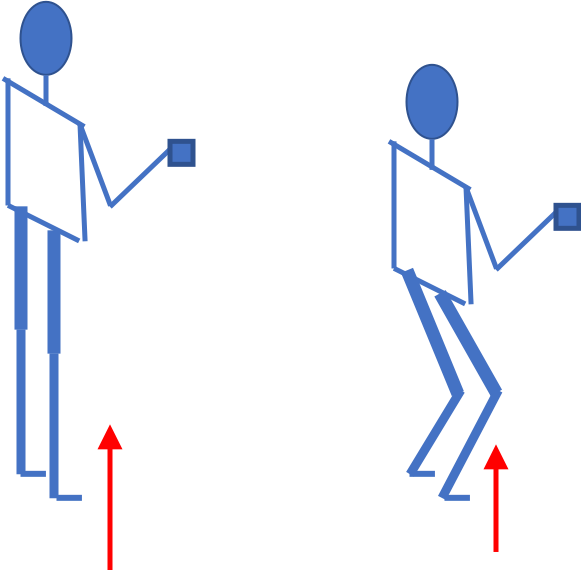(a) First find the impulse on the person from the impact on the ground. Calculate both its magnitude and direction.

(b) Find the average force on the feet if the person keeps his leg stiff and straight and his CM drops by only $1.0\text{ cm}$ vertically and moves $1.0\text{ cm}$ horizontally during the impact. You will need to find the time the impact lasts by making reasonable assumptions about the deceleration. Although the force is not constant during the impact, working with constant average force for this problem is acceptable.

(c) Find the average force on the feet if the person bends his legs throughout the impact so that his CM drops by $50$ cm vertically and moves $5$ cm horizontally during the impact.

(d) Compare the results of part (b) and (c), and draw conclusions about which way is better.

Hint

(a) Use change of momentum along $x$ and $y$ axes. (b) Assume constant acceleration. This would give $\Delta x = v_{av,x} \Delta t\text{.}$ (c) Same as (b) but with different values. (d) You should get (c) to be better.

(a) $617\text{ N.s}, 72^{\circ}$ clockwise from $-x$ axis, (b) $F_x=-29,100\text{ N}\text{,}$ $F_y = 26,590 \text{ N}\text{,}$ $F_\text{av} = 39,000\text{ N}\text{,}$ (c) $F_x=- 5,909\text{ N}\text{,}$ $F_y = 5,318 \text{ N}\text{,}$ $F_\text{av} = 8,000\text{ N}\text{,}$ (d) Bending your knee is better since you reduce the force of impact on you by spreading the impulse over longer interval.

Solution 1 (a)

(a) The impulse on the person is equal to change in his mometum during the impact, i.e., during the time he hits the ground and comes to rest. We have following for $x$ and $y$ components of his velocity instant before impact and when he comes to rest. Here $x$ is horizontal and positive $y$ is vertically up.

\begin{align*} \amp \text{Before: }\ \ v_{x,i} = 3.0\text{ m/s}, \ \ v_{y,i} = -9.0\text{ m/s}, \\ \amp \text{After: }\ \ v_{x,f} = 0, \ \ v_{y,f} = 0. \end{align*}

Therefore, the impulse on the person has the following components.

\begin{align*} J_x \amp = m v_{x,f} - m v_{x,i} = -65\times 3 = -195\text{ N.s}, \\ J_y \amp = m v_{y,f} - m v_{y,i} = -65\times (-9.0) = 585\text{ N.s}. \end{align*}

From these we get the magnitude and the arctangent-angle.

\begin{align*} J \amp = \sqrt{J_x^2 + J_y^2} = 617 \text{ N.s}, \\ \theta \amp = tan^{-1}\left( \dfrac{J_y}{J_x}\right) = tan^{-1}\left( \dfrac{ 585}{-195}\right) = -72^\circ. \end{align*}

Since $(-195, 585)$ is in the second quadrant, the negative angle gives the dfirection as the clockwise angle with respect to the negative $x$axis.

Solution 2 (b)

(b) We assume constant accelerations in the $x$ and $y$ directions as a result of coming to rest within assumed distances. The formulas that will give $\Delta t$ will be

\begin{align*} \Delta x \amp = v_{\text{av},x}\,\Delta t = \dfrac{v_{xi}}{2} \Delta t. \\ \Delta y \amp = v_{\text{av},y}\,\Delta t = \dfrac{v_{yi}}{2} \Delta t. \end{align*}

We will get two different times here

\begin{align*} \Delta t \amp = \dfrac{2\Delta x}{v_{xi}} = \dfrac{2\times 0.01}{3} = 0.0067\text{ s},\\ \Delta t \amp = \dfrac{2\Delta y}{v_{yi}} = \dfrac{2\times 0.01}{9} = 0.0022\text{ s}. \end{align*}

These used with the $x$ and $y$ components of impulse, $J_x$ and $J_y$ will give the components of the force from the floor.

\begin{align*} F_x \amp = -195\text{ N.s} / 0.0067\text{ s} = -29,100\text{ N}. \\ F_y \amp = 585\text{ N.s} / 0.0022\text{ s} = 26,590 \text{ N}. \end{align*}

Therefore, an estimate of the magnitude of the average force from the floor will be

\begin{equation*} F_\text{av} = \sqrt{ 29,100^2 + 26,590^2 } = 39,000\text{ N}. \end{equation*}
Solution 3 (c)

(c) We assume constant accelerations in the $x$ and $y$ directions as a result of coming to rest within assumed distances as above. The formulas that will give $\Delta t$ will again be

\begin{align*} \Delta x \amp = \dfrac{v_{xi}}{2} \Delta t. \\ \Delta y \amp = \dfrac{v_{yi}}{2} \Delta t. \end{align*}

We will get two different times here

\begin{align*} \Delta t \amp = \dfrac{2\Delta x}{v_{xi}} = \dfrac{2\times 0.05}{3} = 0.033\text{ s},\\ \Delta t \amp = \dfrac{2\Delta y}{v_{yi}} = \dfrac{2\times 0.50}{9} = 0.11\text{ s}. \end{align*}

These used with the $x$ and $y$ components of impulse, $J_x$ and $J_y$ will give the components of the force from the floor.

\begin{align*} F_x \amp = -195\text{ N.s} / 0.033\text{ s} = -5,909\text{ N}. \\ F_y \amp = 585\text{ N.s} / 0.0022\text{ s} = 5,318 \text{ N}. \end{align*}

Therefore, an estimate of the magnitude of the average force from the floor will be

\begin{equation*} F_\text{av} = \sqrt{ 5,909^2 + 5,318^2 } = 8,000\text{ N}. \end{equation*}
Solution 4 (d)

(d) Bending your knee is better since you reduce the force of impact on you by spreading the impulse over longer interval.

A chain of mass $M$ and length $L$ is suspended vertically with its bottom touching a pan of mass $m$ on a scale. The chain is then let go from rest. As chain falls, the scale reads the force on the pan needed to balance the weight of the chain that is on the pan and the chain link that is impacting the pan at that instant. Assume each chain link is infinitesimally small and comes to rest instantaneously upon impact so that at each instant the entire momentum of an infinitesimal part of the chain link falling on the pan is transferred to the pan.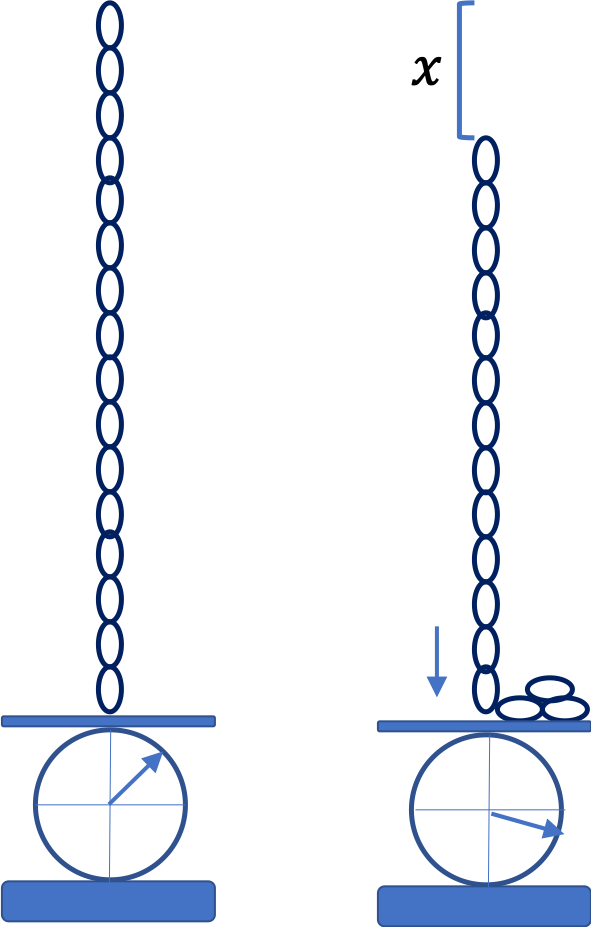(a) Find an expression of the reading of the scale when a length $x$ has fallen.

(b) What is the reading on the scale at the instant the whole chain has fallen to the fan.

(c) What is the reading on the scale after the whole chain has fallen and everything has come to rest?

Hint

Look at momentum change during interval $t$ to $t + \Delta t\text{,}$ where $t$ is the instant when $x$ length has fallen and are already at rest on the pan.

(a) $3 \left( \dfrac{M}{L} \right) x g\text{,}$ (b) $3Mg\text{,}$ (c) $Mg\text{.}$

Solution 1 (a)

(a) We look at the normal force on the pan, which is the reading in the scale, duration $t$ to $t + \Delta t\text{.}$ During this time the chain between $x$ and $x+\Delta x$ strikes the pan and deposits its momentum. The pan already has $m = (M/L)x$ at rest on the pan and another mass $\Delta m = (M/L)\Delta x$ with speed $v = \sqrt{2gx}\text{,}$ due to free fall by distance $x\text{,}$ deposits its momentum. Therefore, the normal force on the pan will be

\begin{equation*} N(t) = \left( \dfrac{M}{L}x\right) g + \left( \dfrac{M}{L} \Delta x \right) \sqrt{2gx}\dfrac{1}{\Delta t}, \end{equation*}

The $\Delta x/\Delta t$ in the second term is just the speed at this instant, which is $v = \sqrt{2gx}\text{.}$ Therefore, we have

\begin{equation*} N(t) = \left(\dfrac{M}{L}x\right) g + \left( \dfrac{M}{L} \right) 2gx = 3 \left( \dfrac{M}{L} \right) x g. \end{equation*}
Solution 2 (b)

Putting $x=L$ in the answer in (a), we get $N = 3Mg$ for the reading at this instant.

Solution 3 (c)

After everything has come to rest, the reading will just be $Mg\text{.}$

A chain of length $L$ rests on a frictionless horizontal table. When a small portion of length $\delta$ of the chain is pushed over the side of the table and let go from rest, the entire chain falls off the table.

(a) What is the length of the chain that has fallen off the table by time $t\text{?}$ (b) What is the speed with which chain is falling?

Hint

Set up $\vec F \Delta t = \Delta \vec p\text{.}$ Also note that external force is weight of only the hanging part.

(a) $\delta\, \cosh( \sqrt{g/L}\,t )\text{,}$ (b) $\delta\,\sqrt{g/L}\, \sinh( \sqrt{g/L}\,t )\text{.}$

Solution

Figure 7.10.66 shows situation at instant $t\text{.}$ At an instant $\Delta t$ later, speed of the entire chain will be $v + \Delta v\text{.}$ Let $\lambda$ be mass per unit length. Noting that external force is only the weight of the hanging part, we will get the following $y$-equation of motion.

\begin{equation*} (v_y+\Delta v_y) \lambda L - v_y \lambda L = \lambda y g \Delta t. \end{equation*}

Here $v_y = dy/dt\text{,}$ which is equal to $v$ in the figure. Dividing by $\Delta t$ and taking the infinitesimal time limit, we get

\begin{equation*} \frac{dv_y}{dt} = \frac{g}{L} y. \end{equation*}

This is same as

\begin{equation*} \frac{d^2y}{dt^2} = \frac{g}{L} y. \end{equation*}

The general solution of this differential equation is

\begin{equation*} y = A e^{\sqrt{g/L}\,t} + B e^{-\sqrt{g/L}\,t}, \end{equation*}

where $A$ and $B$ are constants. To fix them, we will use $y=\delta$ and $v_y=0$ at $t=0\text{.}$ First we get expression for $v_y$ by taking a derivative.

\begin{equation*} v_y = \sqrt{g/L}\, \left( A e^{\sqrt{g/L}\,t} - B e^{-\sqrt{g/L}\,t} \right). \end{equation*}

Thus at $t=0\text{,}$ we have

\begin{align*} \amp A + B = \delta.\\ \amp \sqrt{g/L}\,(A - B) = 0. \end{align*}

Therefore,

\begin{equation*} A = B = \frac{\delta}{2}. \end{equation*}

Hence, we have the following expressions for position and velocity of the bottom of the falling chain.

\begin{align*} \amp y = \delta\, \cosh( \sqrt{g/L}\,t ).\\ \amp v_y = \delta\,\sqrt{g/L}\, \sinh( \sqrt{g/L}\,t ). \end{align*}

A can is placed in an inverted position on a steady stream of water coming out a hose vertically (Figure 7.10.68). Initially, the can is pushed up, but at some height the can becomes suspended in air. Suppose speed of water at the hose is $v_0$ in $\text{m/s}\text{,}$ $V$ $\text{kg/m}^3$ of water flow per second, $\rho$ the density of water in $\text{kg/m}^3\text{,}$ and $M$ the mass of the can in $\text{kg}\text{.}$ What will be the height if we assume that after hitting the can, water hits the can elastically, i.e., water is turned back at the same speed? (Adapted from Kleppner and Kolenkow)

Hint

Balance weight of the can by the force of water on can. Find force of water in can by finding force of can on water in changing the momentum of water.

$\frac{1}{2g}\left[ v_0^2 - \left( \frac{Mg}{2\rho V}\right)^2 \right]\text{.}$

Solution

Strategy: By looking at the rate of change of momentum of water, we will get the force exerted by the can on water. By third law, water will be exerting the same magnitude force on the can. Then, we will balance forces on the can to find the necessary condition for the problem.

Impulse on water by the can will equal the rate at which momentum of water is changed by hitting the can. Let $m$ denote the mass of water that strikes the can in duration $\Delta t\text{.}$ Let $F$ be the magnitude of force between can and water. Let positive $y$-axis be pointed up in Figure 7.10.68.

\begin{equation*} -F \Delta t = m (-v) - m v = - 2 mv. \end{equation*}

Here $v$ is the speed with which water hits the can. Since water had vertical velocity $v_0$ a height $h$ below the can, we have

\begin{equation*} v = \sqrt{v_0^2 - 2 g h}. \end{equation*}

And $m$ will be

\begin{equation*} m = \rho V \Delta t. \end{equation*}

Therefore, the magnitude of force by water on the can will be

\begin{equation*} F = 2\rho V \sqrt{v_0^2 - 2 g h}. \end{equation*}

Now, this force balances the weight of the can to keep the can suspended. Therefore,

\begin{equation*} 2\rho V \sqrt{v_0^2 - 2 g h} = Mg. \end{equation*}

Therefore,

\begin{equation*} h = \frac{1}{2g}\left[ v_0^2 - \left( \frac{Mg}{2\rho V}\right)^2 \right]. \end{equation*}